# InfoQ 极客传媒 15 周年庆征文|海王的鱼塘是怎样炼成的

• 2022 年 6 月 17 日
• 本文字数：4019 字

阅读完需：约 13 分钟## 一、引言

Flocking algorithm 国内一般称为蜂拥算法，由许多离散的动物形成，但群体整体上是流动的，这是个体行为的综合结果。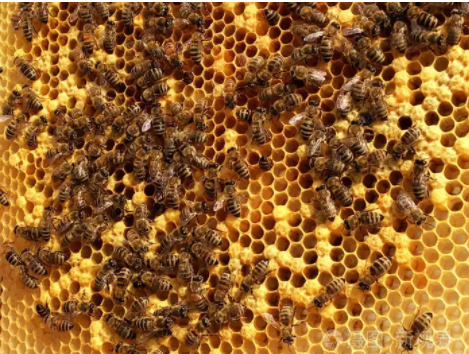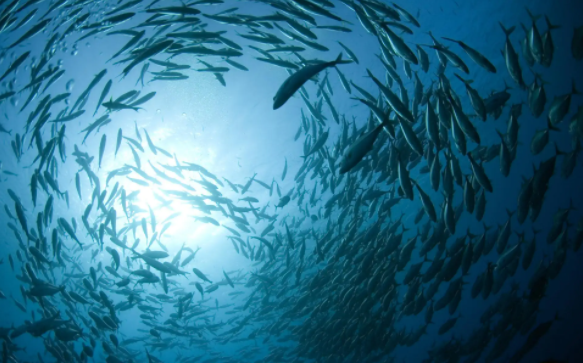## 二、发展1987 年 7 月，Craig Reynolds这位老先生率先提出了经典的 Flocking 模型，该模型要求群体行为满足三个规则：

• 聚合：独立的个体逐渐加入到群体

• 速度匹配：个体与群体的航向保持一致，不要脱离

• 分离：避免群体内的个体相互碰撞

## 三、鱼群

### 1.组件

    [Header("Fish Setting")]//控制面板    [Range(0.0f,5.0f)]    public float min;//速度最小值    [Range(0.0f, 5.0f)]    public float max;//速度最小值    [Range(1.0f, 10.0f)]    public float neighborDistance;//聚合的距离    [Range(0.0f, 5.0f)]    public float RotationSpeed;//转速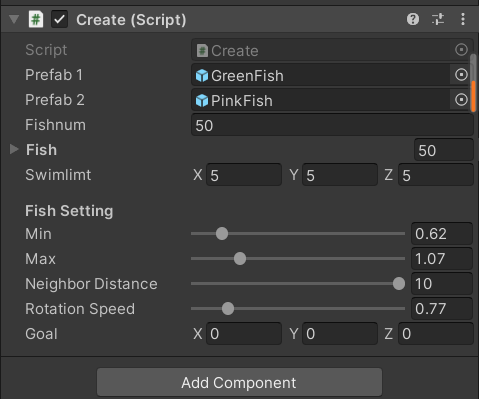### 2.生成鱼群

    public GameObject prefab1;//🐟种类1    public GameObject prefab2;//🐟种类2    public int fishnum=50;//初始化🐟数量    public GameObject[] fish;//数组存储    public Vector3 swimlimt = new Vector3(5, 5, 5);//边界10*10*10

    public void Start()    {        fish = new GameObject[fishnum];        for(int i=0;i<fishnum;i++)        {            Vector3 pos = this.transform.position + new Vector3(Random.Range(-swimlimt.x,swimlimt.x),                                                                Random.Range(0, swimlimt.y),                                                                Random.Range(-swimlimt.z, swimlimt.z));            if(i%2==0)                fish[i] = (GameObject)Instantiate(prefab1,pos,Quaternion.identity);//克隆🐟            else                fish[i] = (GameObject)Instantiate(prefab2, pos, Quaternion.identity);            fish[i].GetComponent<FlockSpeed>().sp = this;//两个脚本间联系        }    }

### 3.鱼群运动

    private void Update()    {        speed = Random.Range(sp.min, sp.max);//速度范围        this.transform.Translate(0, 0, speed * Time.deltaTime);//开始移动      }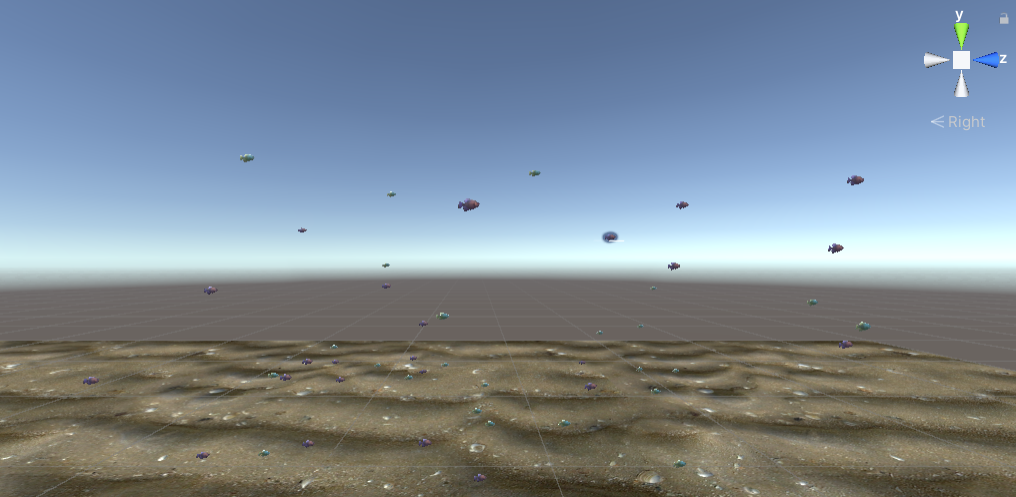## 四、聚合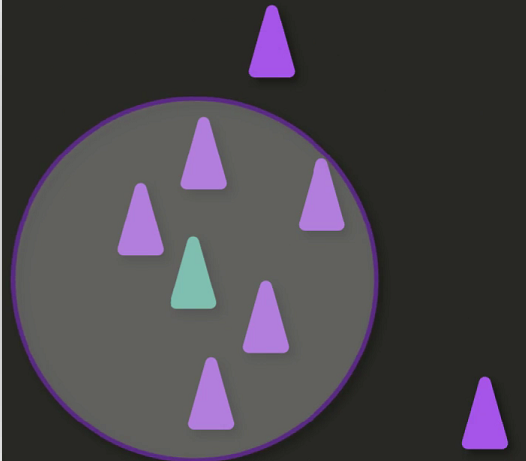平均位置=鱼群位置相加的总和/鱼群的数量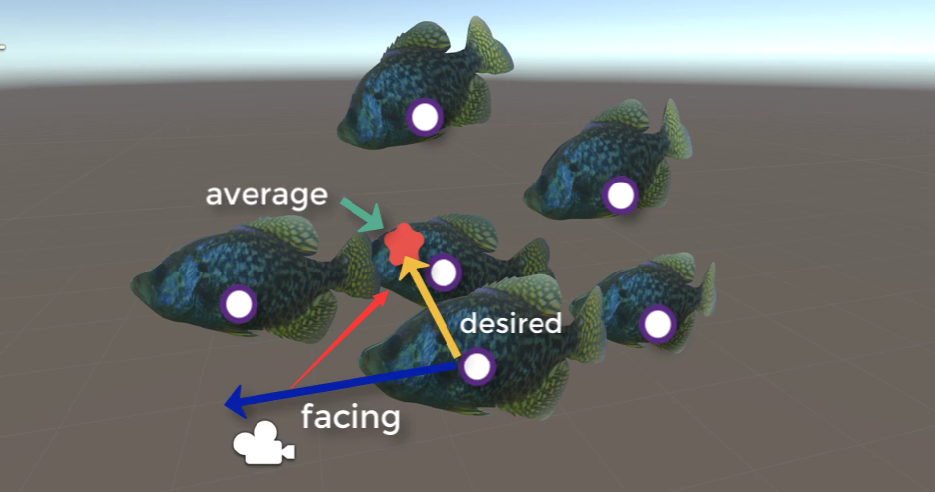average-desired

void Community()    {        GameObject[] gos=sp.fish;        float Distance;        Vector3 center = Vector3.zero;        Vector3 avoid = Vector3.zero;        int size=0;        float goSpeed=0.01f;        foreach(GameObject go in gos)        {            if(go!=this.gameObject)//遍历数组内除掉本身所有的鱼            {                Distance = Vector3.Distance(this.transform.position, go.transform.position);//相邻两条鱼距离                if (Distance<=sp.neighborDistance)//满足集群规则                {                    size++;                    center+= go.transform.position;                    if (Distance < limt)//避免碰撞                    {                        avoid += this.transform.position - go.transform.position;                    }                    FlockSpeed flockSpeed = go.GetComponent<FlockSpeed>();                    goSpeed += flockSpeed.speed;                }            }        }        if(size>0)        {            center = center / size+(sp.goal- this.transform.position );//后面加上的是目标位置，朝着目标移动            goSpeed = goSpeed / size;//平均速度            Vector3 direction = (center + avoid) - transform.position;            if(direction!=Vector3.zero)//需要转向            {                transform.rotation = Quaternion.Slerp(transform.rotation, Quaternion.LookRotation(direction), sp.RotationSpeed * Time.deltaTime);//from to 平滑旋转  Quaternion.LookRotation注视旋转            }        }    }

## 五、速度匹配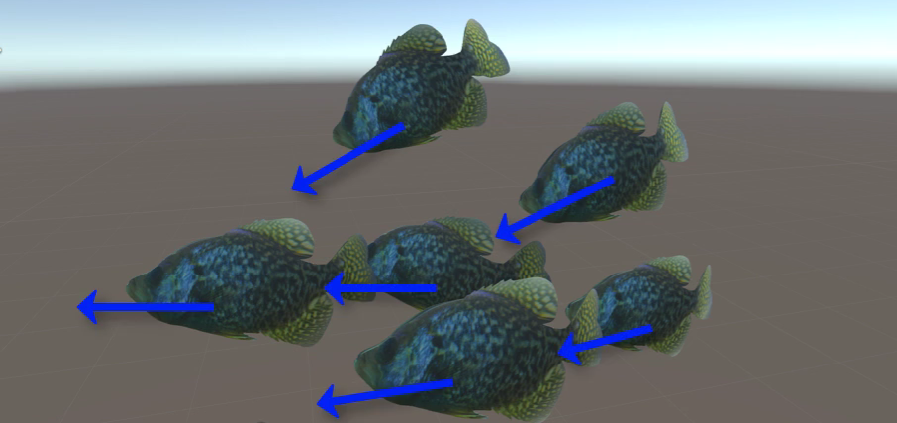虚拟领航者的速度=总体速度和/鱼群数目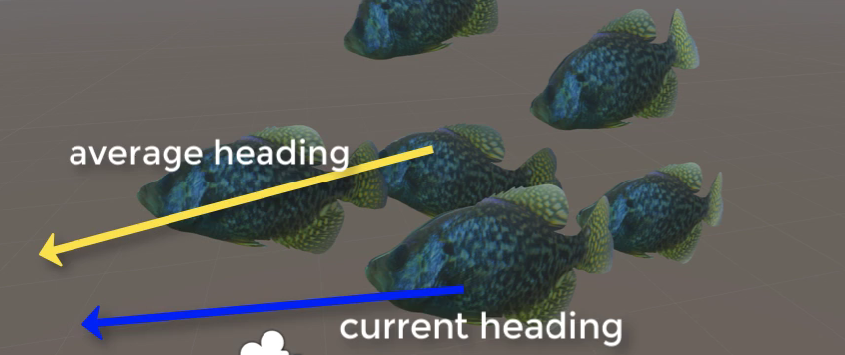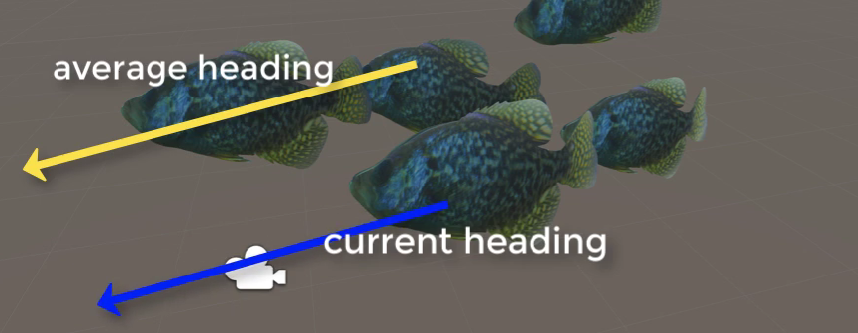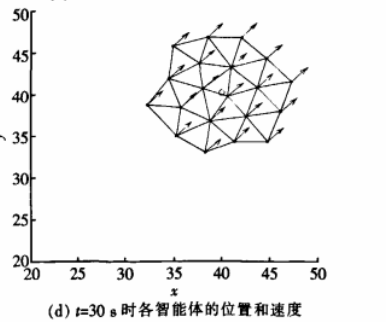## 六、捕食

private void Update()    {        //10%的几率更换目标        if(Random.Range(0,100)<10)            goal = this.transform.position + new Vector3(Random.Range(-swimlimt.x, swimlimt.x),                                                         Random.Range(0, swimlimt.y),                                                         Random.Range(-swimlimt.z, swimlimt.z));    }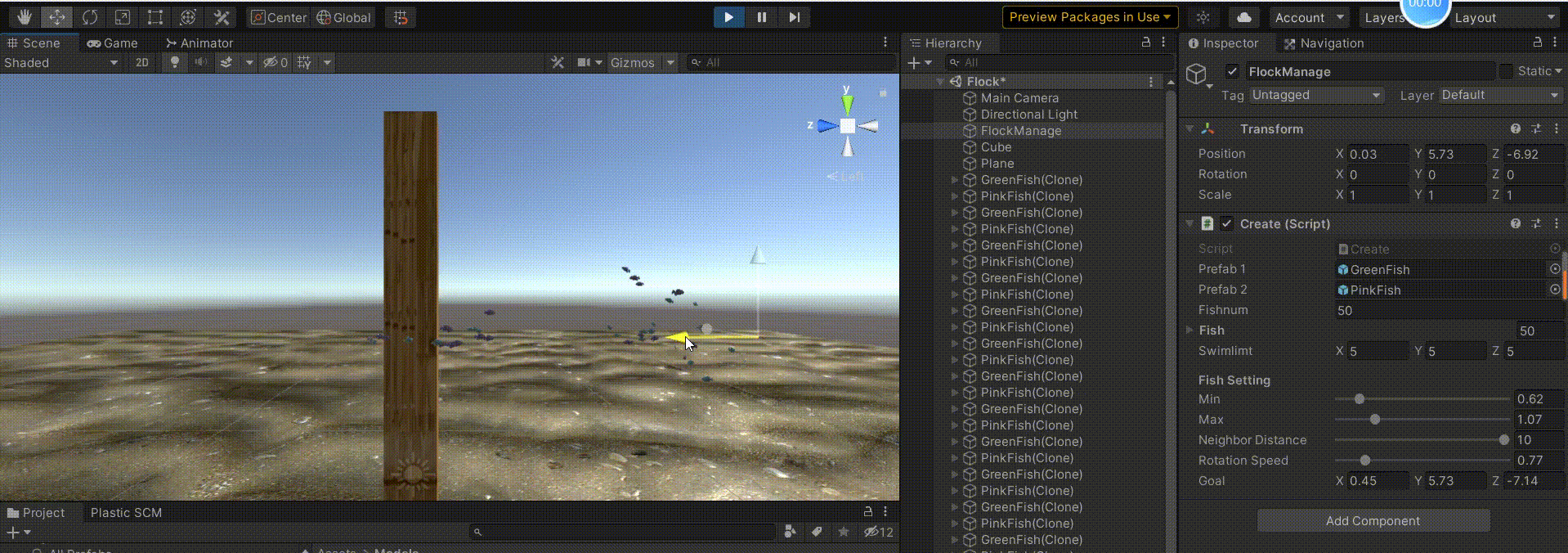## 七、分离

### 1.躲避🐟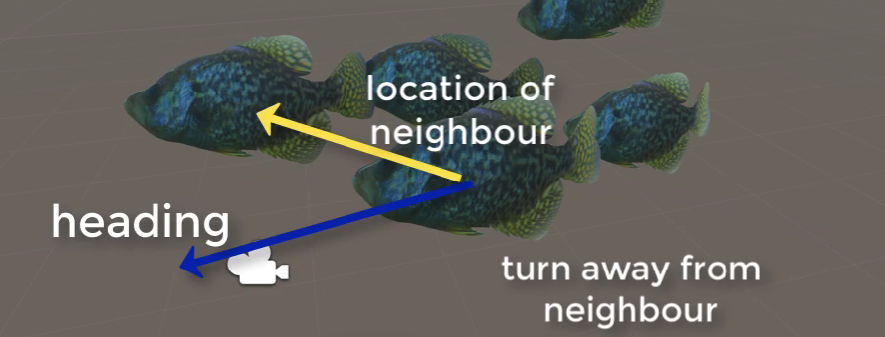🐟和🐟之间的躲避变量是avoid，代码部分也在上面的 Community()函数里面了。

### 2.躲避边界

        Bounds b = new Bounds(sp.transform.position,sp.swimlimt);//边界的盒子        Vector3 direction = Vector3.zero;        RaycastHit hit = new RaycastHit();        if (!b.Contains(transform.position))//即将超出边界        {            turning = true;//开始转向            direction = sp.transform.position - transform.position;//转向方向指向中心位置        }          else            turning = false;

### 3.躲避障碍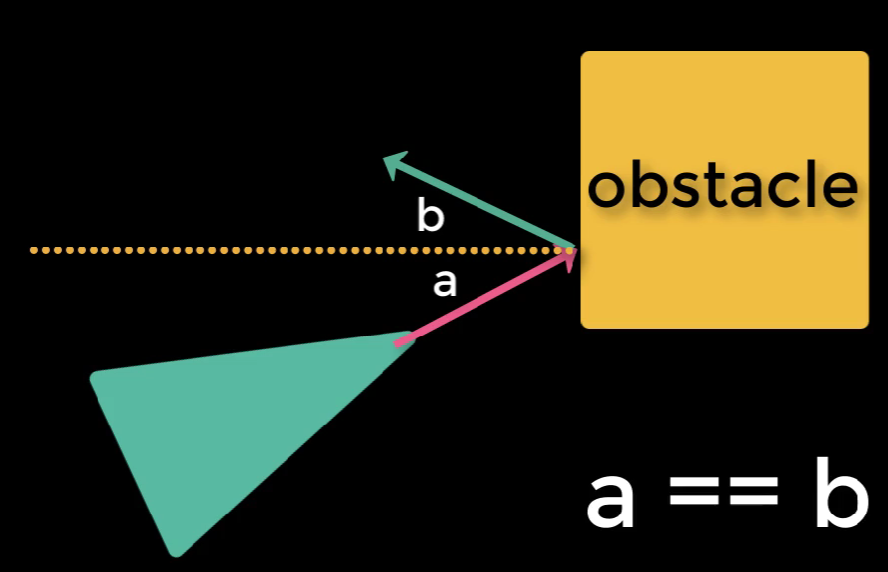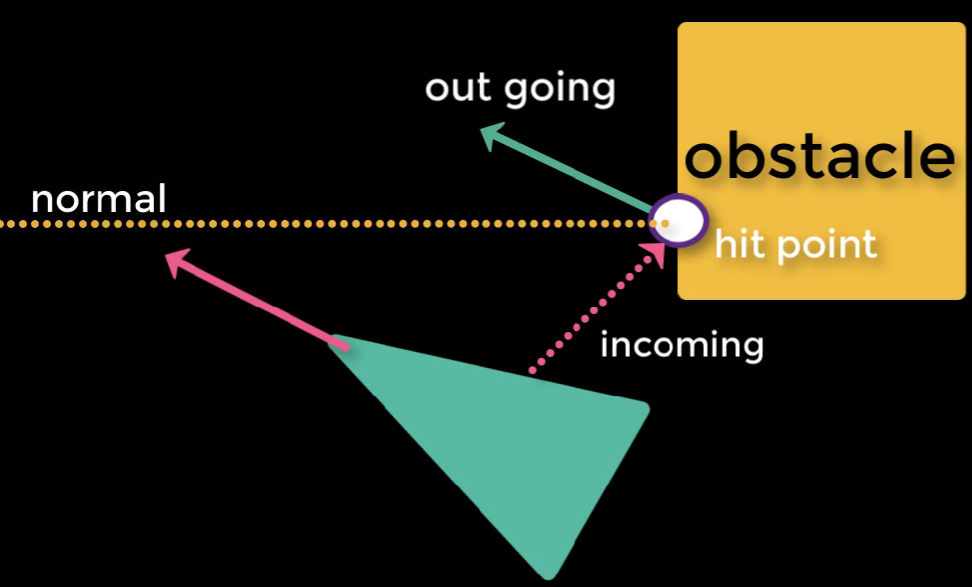if(Physics.Raycast(this.transform.position,transform.forward*5,out hit))//遇到障碍物        {            //Debug.DrawRay(this.transform.position,this.transform.forward*5,Color.red);红色射线            direction = Vector3.Reflect(this.transform.forward, hit.normal);//反射角 = 入射角 转向            turning = true;        }

        if(turning)        {            this.transform.rotation = Quaternion.Slerp(transform.rotation,             Quaternion.LookRotation(direction), sp.RotationSpeed * Time.deltaTime);          }        else        {              Community();//聚合函数        }

## 九、总结

Flocking 算法是群居动物的合作行为，通过个体间的相互作用实现整体的目标。本文实现的只是一个简单的🐟集群模拟，仅仅是模式识别领域数据聚类的一个算法分支，转向角度、视野、领队方面还没有实现。

【参考资料】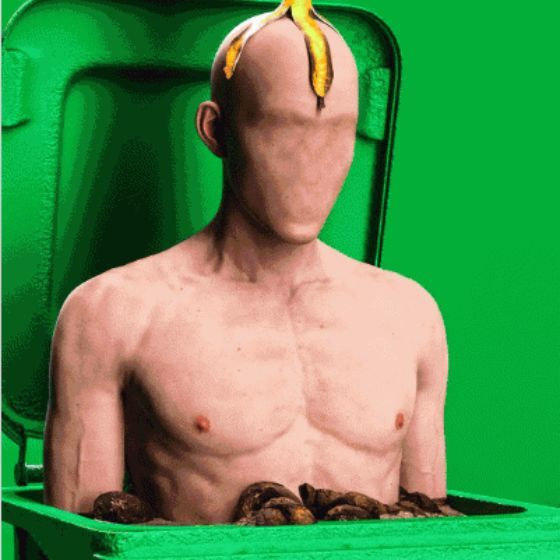## 评论 (1 条评论)2022 年 06 月 17 日 20:31